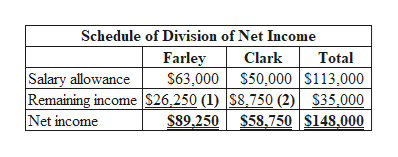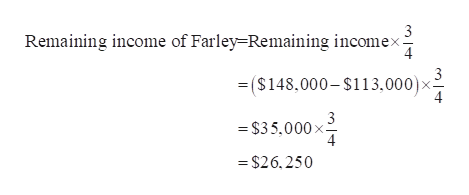# Dividing LLC IncomeMartin Farley and Ashley Clark formed a limited liability company with an operating agreement that provided a salary allowance of \$63,000 and \$50,000 to each member, respectively. In addition, the operating agreement specified an income-sharing ratio of 3:1. The two members withdrew amounts equal to their salary allowances. Revenues were \$668,000 and expenses were \$520,000, for a net income of \$148,000.a. Determine the division of \$148,000 net income for the year.Schedule of Division of Net Income FarleyClarkTotalSalary allowance\$\$\$Remaining income Net income\$\$\$b. Provide journal entries to close the (1) revenues and expenses and (2) drawing accounts for the two members. For a compound transaction, if an amount box does not require an entry, leave it blank.(1)            (2)           c. If the net income were less than the sum of the salary allowances, how would income be divided between the two members of the LLC?If the net income of the LLC were less than the sum of the salary allowances, members would still be credited with their salary allowances. The difference between the net income and total salary allowances would be allocated to each partner as , according to the ratio.

Question
232 views

Dividing LLC Income
Martin Farley and Ashley Clark formed a limited liability company with an operating agreement that provided a salary allowance of \$63,000 and \$50,000 to each member, respectively. In addition, the operating agreement specified an income-sharing ratio of 3:1. The two members withdrew amounts equal to their salary allowances. Revenues were \$668,000 and expenses were \$520,000, for a net income of \$148,000.
a. Determine the division of \$148,000 net income for the year.
Schedule of Division of Net Income

Farley
Clark
Total
Salary allowance
\$
\$
\$
Remaining income

Net income
\$
\$
\$
b. Provide journal entries to close the (1) revenues and expenses and (2) drawing accounts for the two members. For a compound transaction, if an amount box does not require an entry, leave it blank.
(1)

(2)

c. If the net income were less than the sum of the salary allowances, how would income be divided between the two members of the LLC?
If the net income of the LLC were less than the sum of the salary allowances,

members would still be credited with their salary allowances. The difference between the net income and total salary allowances would be allocated to each partner as

, according to the

ratio.

check_circle

Step 1

a.

Determine the division of \$148,000 net income for the year.help_outlineImage TranscriptioncloseSchedule of Division of Net Income Farley \$63,000 \$50,000 S113,000 Clark Total Salary allowance Remaining income S26,250 (1) S8,750 (2) Net income \$35,000 S89,250 S58.750 S148.000 fullscreen
Step 2

Working Note (1):

Calculate the remaining income of Farley.help_outlineImage Transcriptionclose3 Remaining income of Farley=Remaining incomex. 4 3 = (\$ 148,000- \$113,000): 4 =\$35,000x 4 =\$26.250 fullscreen
Step 3

Working Note (2):

Calculate the remaining income of Cl...

### Want to see the full answer?

See Solution

#### Want to see this answer and more?

Solutions are written by subject experts who are available 24/7. Questions are typically answered within 1 hour.*

See Solution
*Response times may vary by subject and question.
Tagged in